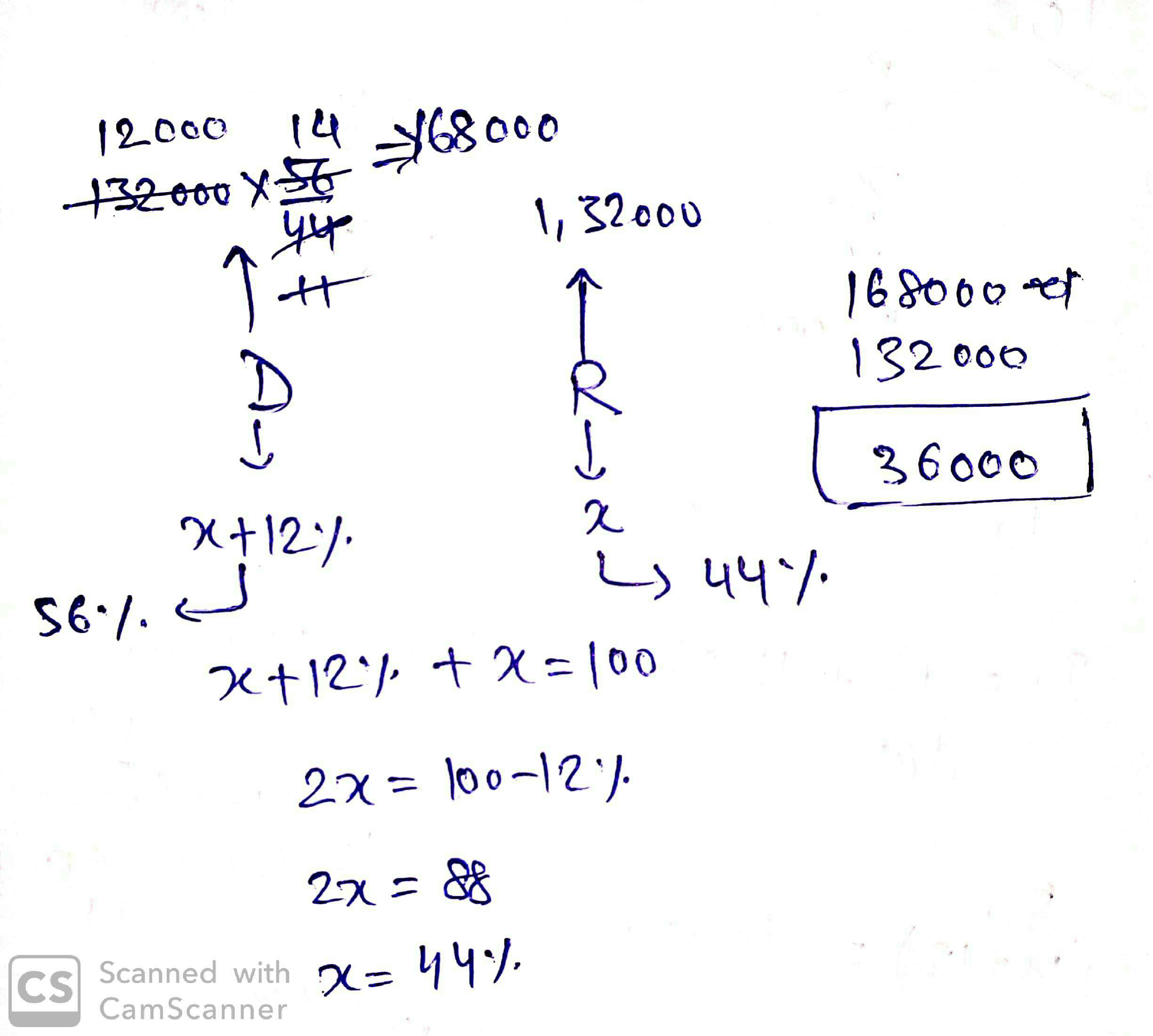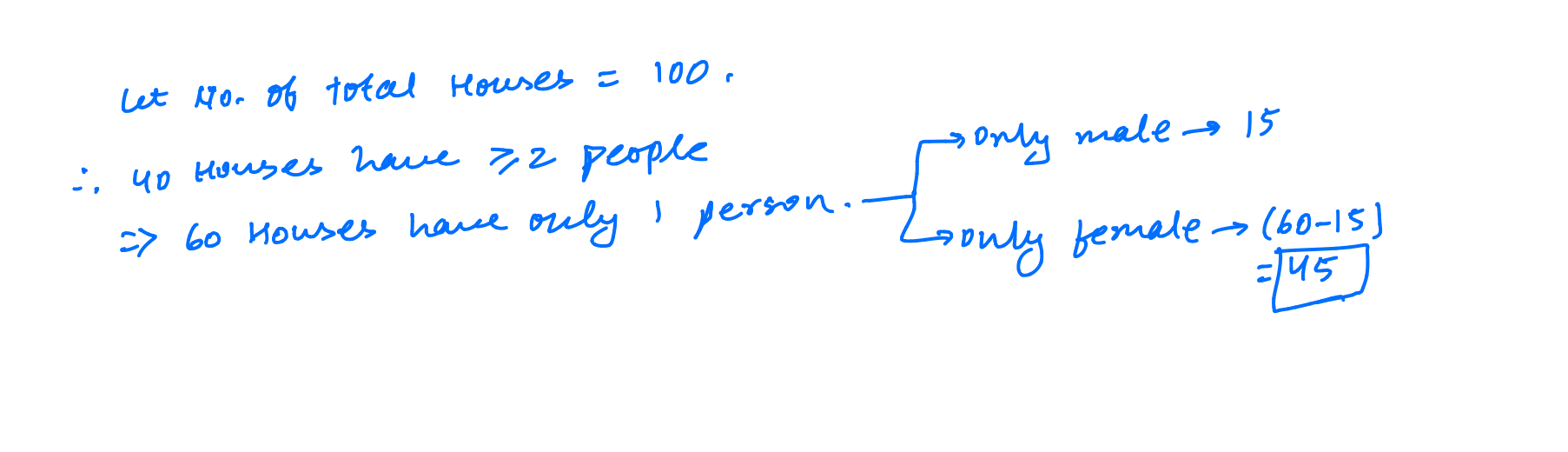CSAT 2021 Practice Questions - ForumIAS

# CSAT 2021 Practice Questions

Will be posting some csat questions for practice here on a daily basis!Neyawn,Integerand12 otherslike this
32k views

8. The difference between the value of a number increased by 12.5% and the value of the original number decreased by 25% is 30. What is the original number?

A. 60

B. 80

C. 40

D. 120

1.3k views

8. The difference between the value of a number increased by 12.5% and the value of the original number decreased by 25% is 30. What is the original number?

A. 60

B. 80

C. 40

D. 120

B:

1.125x - 0.75x= 30

=>x= 80musa,GaryVee
2k views

8. The difference between the value of a number increased by 12.5% and the value of the original number decreased by 25% is 30. What is the original number?

A. 60

B. 80

C. 40

D. 120

B:

1.125x - 0.75x= 30

=>x= 80

Correct.

1.3k views1.5k views36000 is correct.

1.3k views

A bag contains 20 balls. 8 balls are green, 7 are white and 5 are red. What is the minimum number of balls that must be picked up from the bag blindfolded (without replacing any of it) to be assured of picking at least one ball of each colour?

a. 13      b. 11      c. 17      d. 16GaryVee,
1.5k views

A bag contains 20 balls. 8 balls are green, 7 are white and 5 are red. What is the minimum number of balls that must be picked up from the bag blindfolded (without replacing any of it) to be assured of picking at least one ball of each colour?

a. 13      b. 11      c. 17      d. 16

16.

If we pick up 5 it can be only gree or all red or all white. But if we choose 6, then even if all 5 red balls are picked, we know that at least one ball will be gree or white.

If we pick up 8, we know that all 8 balls may be gree only

If we pick up 8 balls, which by our bad luck is all green, and then we keep picking up balls and by bad luck they can be all white balls till 15 balls. but 16th ball will 100% be of red color because all other balls have been picked up.

good questions. earlier when i saw this question, i thought it was pwrmutationr or probability. then i thought i will solve it on pen and paper, when i sat down, it was simpler!Naadan_Parinda,GaryVeeand1 otherslike this
2.1k views

A selection is to be made for one post of Principal and two posts of Vice-Principal. Amongst the six candidates called for the interview, only two are eligible for the post of Principal while they all are eligible for the post of Vice-Principal. The number of possible combinations of selectees is

a. 4    b. 12       c. 18       d. None of the above

1.6k views

A selection is to be made for one post of Principal and two posts of Vice-Principal. Amongst the six candidates called for the interview, only two are eligible for the post of Principal while they all are eligible for the post of Vice-Principal. The number of possible combinations of selectees is

a. 4    b. 12       c. 18       d. None of the above

Is it 6c2 * 2c1

Have forgotten the formula :P

1.1k views
Can you explain the logic?
@Nikita should be 5c2*2c1

1.1k views
@Nikita 2c1 for princi because 1  will be selected from 2 candidates.
5c2 for VP  because 1 person  is already selected for princi,rest 2 would be selected from remaining 5 candidatesgoldberry,
1k views
Thank you. I can never understand these questions. But thanks for the help.
@Nikita 2c1 for princi because 1  will be selected from 2 candidates.
5c2 for VP  because 1 person  is already selected for princi,rest 2 would be selected from remaining 5 candidates

1.1k views

19. In a recent survey 40% houses contained two or more people. Of those houses containing only one person 25% were having only a male. What is the percentage of all houses which contain exactly one female and no males (Assume that each house contains at least one person)?

(a) 60

(b) 40

(c) 45

(d) 75

1.3k views

19. In a recent survey 40% houses contained two or more people. Of those houses containing only one person 25% were having only a male. What is the percentage of all houses which contain exactly one female and no males (Assume that each house contains at least one person)?

(a) 60

(b) 40

(c) 45

(d) 75

C. 451.1k views

19. In a recent survey 40% houses contained two or more people. Of those houses containing only one person 25% were having only a male. What is the percentage of all houses which contain exactly one female and no males (Assume that each house contains at least one person)?

(a) 60

(b) 40

(c) 45

(d) 75

C. 45Right!eclectus,
2.2k views

20. Set A contains all the even numbers between 2 and 50 inclusive. Set B contains all the even numbers between 102 and 150 inclusive. What is the difference between the sum of elements of set B and that of set A?

a. 2500

b. 5050

c. 11325

d. 6275

1.3k views

20- a

No. Of terms between 2 & 50= 25.

Sum= (102-2) + (104-4) + .......+ (150-50)

=>Sum= 100*25

=2500

1.1k views

20- a

No. Of terms between 2 & 50= 25.

Sum= (102-2) + (104-4) + .......+ (150-50)

=>Sum= 100*25

=2500

Correct.eclectus,
2.2k views

21. When the digits of two-digit numbers are reversed, the number increases by 27, the sum of such two-digit numbers

(a) 235

(b) 249

(c) 213

(d) 180

1.3k viewsWrite your comment…